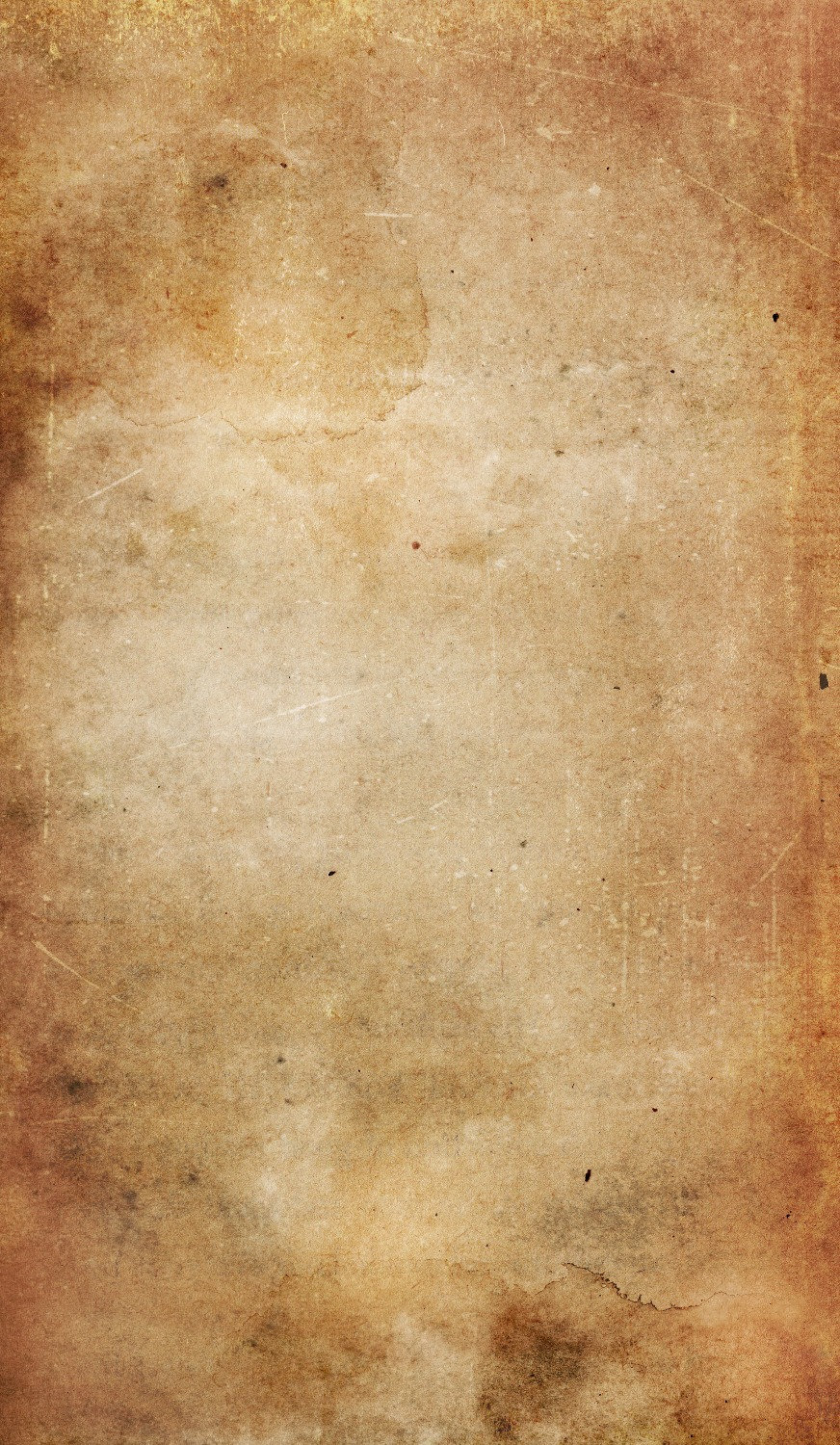Search

# How To Paint - Aftermath Grumple

Here's a quick tutorial on how I painted the hero Grumple for Aftermath. You can find tutorials for the other heroes as well as the minions here.

## Recipe

• Formula P3 Battlefield Brown

• Formula P3 Gun Corps Brown

• Formula P3 Meaty Ochre

• Formula P3 Menoth White Base

• Formula P3 Menoth White Highlight

• Formula P3 Moldy Ochre

• Formula P3 Khardic Flesh

• Formula P3 Midlund Flesh

• Formula P3 Ryn Flesh

• Formula P3 Ironhull Grey

• Formula P3 Underbelly Blue

• Formula P3 Frostbite

• Formula P3 Morrow White

• Formula P3 Bloodtracker Brown

• Formula P3 Bogrin Brown

• Formula P3 Jack Bone